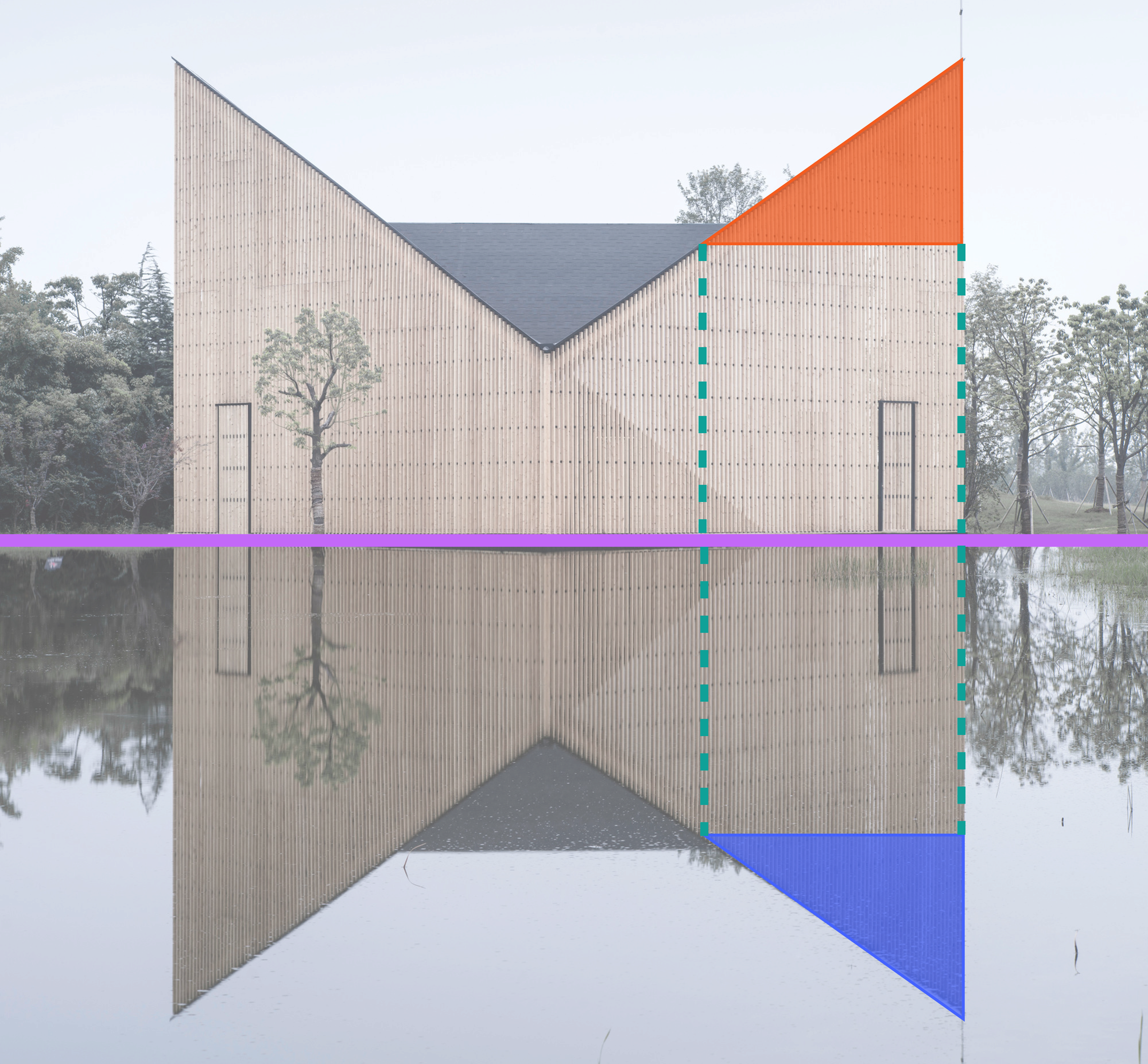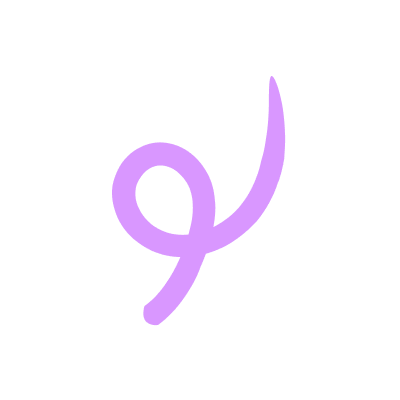IntroductionIntroduction# Reflections

INTRO
A reflection is a type of rigid transformation, which means it changes the position or orientation of an image without changing its size or shape.

A reflection does this by flipping an image across a fixed line - just like a mirror!The fixed line is called the line of reflection, and in the image below, it’s where the ground meets the water. Notice that the points from the actual building and the points from the reflection of the building are equal distances away from the line of reflection on opposite sides.In this example, the line of reflection is horizontal, but it can also be vertical or diagonal.
Check out our
Calculator
or explore our
Lesson
and
Practice

CALCULATOR

## Reflection Calculator

### Step 1. Identify the original points.

How many points does your shape have?
KEY STEPS

## How to Do Reflections

### Step 2. Identify the line of reflection.

This is important because when you reflect your original shape over this line, the new shape will still be the same distance from the line in the opposite direction.

### Step 3. Find the distance between each original point and the line of reflection.

Count the number of units from each original point to the line of reflection.

LESSON
Reflections

## Reflections

A reflection works just like a mirror. If we look into a mirror, we’re going to see ourselves looking right back at us.And if we walk closer or farther away from the mirror, our reflection does too.
Similarly, when we reflect a shape across a line of reflection, the new shape is just the original shape flipped over on the opposite side of the line an equal distance away.
If the line of reflection has an equation in the form of then the line is vertical.Even though the line of reflection can change, we can still follow the same steps to complete a reflection.
For each original point, we figure out how far the point is from the line of reflection and place a new point the same distance away on the opposite side of the line of reflection.

### Reflection Formulas

Sometimes it's hard to count the units or diagonals - in those cases, there are a few formulas that can help.
If the line of reflection is vertical like , then each point updates to:
If the line of reflection is horizontal like , then each point updates to:
If the line of reflection is , then each point will just flip:
if the line of reflection is , then each point will flip and negate:
If the line of reflection is a different diagonal and you're not able to count diagonal units, then the math gets way more complicated. If you want help with this, let us know by sending us feedback!
Let’s walk through some examples to see this in action!
PRACTICE
Reflections

## Practice: Reflections

Question 1 of 10: Reflect the following shape across the line .

### Step 1. Identify the original points.

Enter the original points:
 Original Point Point 1 Point 2 Point 3

CONCLUSION
Nice work, look at you go! Thanks for checking out this lesson ☺️🙏. Where to next?Leave Feedback